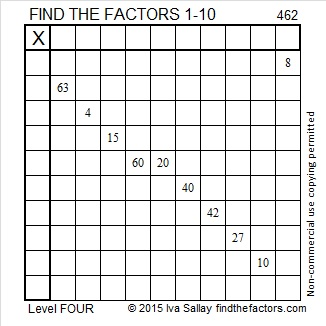# 462 and Level 4

462 is the sum of consecutive prime numbers two different ways. Check the comments to see what those ways are.

Divisibility tricks:

• 462 is even, so it is divisible by 2.
• The sum of the odd numbered digits, 4 + 2 is 6, which is the 2nd digit, so 462 is divisible by 11.
• Since both of those 6’s above are divisible by 3, then 462 is divisible by 3.
• Separate the last digit from the rest and double it. 462 → 46 and 2; Doubling 2, gives us 4. Now subtract that 4 from the remaining digits: 46 – 4 = 42 which is divisible by 7, so 462 is divisible by 7.

Since 462 = 21 × 22, we know that it is two times the 21st triangular number, and it is the sum of the first 21 even numbers.

• 2(1 + 2 + 3 + 4 + 5 + 6 + 7 + 8 + 9 + 10 + 11 + 12 + 13 + 14 + 15 + 16 + 17 + 18 + 19 + 20 + 21) = 462
• 2 + 4 +  6 + 8 + 10 + 12 + 14 + 16 + 18 + 20 + 22 + 24 + 26 + 28 + 30 + 32 + 34 + 36 + 38 + 40 + 42 = 462Print the puzzles or type the solution on this excel file:  10 Factors 2015-04-13

—————————————————————————————————

• 462 is a composite number.
• Prime factorization: 462 = 2 x 3 x 7 x 11
• The exponents in the prime factorization are 1, 1, 1, and 1. Adding one to each and multiplying we get (1 + 1)(1 + 1)(1 + 1)(1 + 1) = 2 x 2 x 2 x 2 = 16. Therefore 462 has exactly 16 factors.
• Factors of 462: 1, 2, 3, 6, 7, 11, 14, 21, 22, 33, 42, 66, 77, 154, 231, 462
• Factor pairs: 462 = 1 x 462, 2 x 231, 3 x 154, 6 x 77, 7 x 66, 11 x 42, 14 x 33, or 21 x 22
• 462 has no square factors that allow its square root to be simplified. √462 ≈ 21.4942—————————————————————————————————## 2 thoughts on “462 and Level 4”

1.abyssbrain

Haha, got lucky there, found it very quickly 🙂

67 + 71 + 73 + 79 + 83 + 89 = 462

•ivasallay

Good for you! I’m glad there is more competition to see who finds them first! 229 + 233 are also consecutive prime numbers that equal 462.

This site uses Akismet to reduce spam. Learn how your comment data is processed.